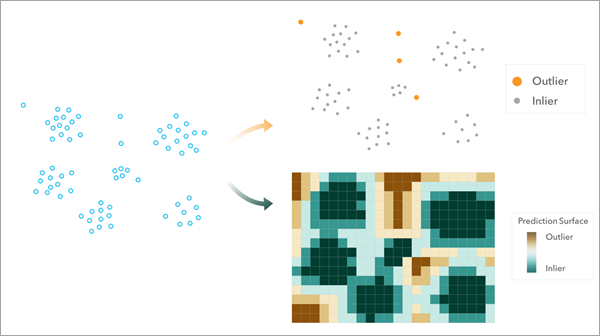# Spatial Outlier Detection (Spatial Statistics)

## Summary

Identifies spatial outliers in point features by calculating the local outlier factor (LOF) of each feature. Spatial outliers are features in locations that are abnormally isolated, and the LOF is a measurement that describes how isolated a location is from its local neighbors. A higher LOF value indicates higher isolation. The tool can also be used to produce a raster prediction surface that can be used to estimate if new features will be classified as outliers given the spatial distribution of the data.

## Illustration## Usage

• This tool identifies points supplied in the Input Features parameter as either spatial outliers or spatial inliers.

• The tool performs an LOF calculation to estimate the degree to which a point feature is an outlier based on the spatial distribution of features in its vicinity.

• The tool uses a local neighborhood around each feature, specified in the Number of Neighbors parameter.

• The Percent of Locations Considered Outliers parameter is used to establish a threshold for the LOF to designate each point feature as an outlier or inlier.

##### Note:

Small differences in values for the Percent of Locations Considered Outliers parameter may result in the same count of output features designated as outliers. This can occur when similarities in spatial distribution for features result in the same LOF value for multiple features.

• The output features include two charts. The first is a bar chart displaying counts of outliers and inliers. The second is a histogram displaying the distribution of LOF values for all point features and the LOF threshold used to determine whether a feature is an outlier or an inlier.

• If the input features have the Shape.Z geometry attribute, the tool will honor the 3D nature of your data by detecting spatial outliers in 3D space. When added to a scene view, the output features display in 3D to visualize the 3D spatial outliers. If the unit (for example, meters) of Shape.Z is not defined in a vertical coordinate system, the unit is assumed to be the same as Shape.X and Shape.Y.

• The Output Prediction Raster parameter is an optional output that contains the LOF result as a continuous surface across the study area that can be used to determine whether future observations are outliers without needing to recalculate the LOF value of the new point. To create the output raster, the input features are used as training data, and the LOF values are calculated at the center of every raster cell based on the spatial distribution of the training data. This output can only be created for 2D input features.

##### Note:

The LOF values of the points will not match the LOF values of the raster cells under each point, even if the points coincide with a cell center of the raster. This is because the feature does not use itself as a neighbor, but the raster cell does use the feature as a neighbor, so each calculation uses different neighbors and produces a different LOF value.

• Breunig, M. M., Kriegel, H. P., Ng, R. T., Sander, J. (2000). "LOF: identifying density-based local outliers." Proceedings of the 2000 ACM SIGMOD international conference on Management of data. (pp. 93-104).

## Syntax

`arcpy.stats.SpatialOutlierDetection(in_features, output_features, {n_neighbors}, {percent_outlier}, {output_raster})`
 Parameter Explanation Data Type in_features The point features used to build the spatial outlier detection model. Each point will be classified as an outlier or inlier based on its local outlier factor. Feature Layer output_features The output feature class containing the local outlier factor for each input feature as well as an indicator of whether the point is a spatial outlier. Feature Class n_neighbors(Optional) The number of neighbors to include when calculating the local outlier factor. The closest features to the input point are used as neighbors. The default is 20. Long percent_outlier(Optional) The percent of locations to be identified as spatial outliers by defining the threshold of the local outlier factor. If no value is specified, a value is estimated at run time and is displayed as a geoprocessing message. Double output_raster(Optional) The output raster containing the local outlier factors at each cell, which is calculated based on the spatial distribution of the input features. Raster Dataset

## Code sample

SpatialOutlierDetection example 1 (Python window)

The following Python window script demonstrates how to use the SpatialOutlierDetection tool.

``````arcpy.stats.SpatialOutlierDetection("Transaction_Locations",
"Transactions_SpatialOutliers", 20, 5,
"Transactions_OutliersPredictionSurface")``````
SpatialOutlierDetection example 2 (stand-alone script)

The following stand-alone Python script demonstrates how to use the SpatialOutlierDetection tool.

``````# Import system modules.
import arcpy

try:
# Set the workspace and input features.
arcpy.env.workspace = 'C:\\SpatialOutlierDetection\\MyData.gdb'
inputFeatures = "PM25_AirQualityStations"

# Set the name of the output features
outputFeatures = "AirQualityStations_SpatialOutliers"

# Set the number of neighbors
numberNeighbors = 8

# Set the percentage of locations considered outliers
pcntLocationsAsOutliers = 10

# Set the output prediction raster
outputPredictionRaster = airQualityStations_OutPredictionRaster

# Run the Spatial Outlier Detection tool
arcpy.stats.SpatialOutlierDetection(inputFeatures, outputFeatures,
numberNeighbors, pcntLocationsAsOutliers, outputPredictionRaster)

except arcpy.ExecuteError:
# If an error occurred when running the tool, print the error message.
print(arcpy.GetMessages())``````

## Environments

Cell Size

This environment only impacts the output raster.

This environment only impacts the output raster.

Snap Raster

This environment only impacts the output raster.

Extent

This environment only impacts the output raster.

## Licensing information

• Basic: Limited
• Standard: Limited